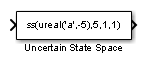Uncertain State Space

• Library:
• Robust Control Toolbox

•Description

The Uncertain State Space block lets you model parametric and dynamic uncertainty in Simulink®. The block accepts uncertain state space (uss) models or any model that can be converted to uss, such as umat, ureal, umargin, and ultidyn objects.

Ports

Input

expand all

For a single-input uncertain system, the input signal is a scalar. For multiple-input systems, combine the system inputs into a vector signal, using blocks such as:

Output

expand all

For a single-output uncertain system, the output signal is a scalar. For multiple-output systems, the output signal is a vector. To split system outputs into scalar signals, use blocks such as:

Parameters

expand all

Specify the uncertain model to simulate as uss object using one of the following:

• Function or expression that evaluates to an uss object. For example:

• ss(ureal('a',-5),5,1,1)

• wt*input_unc, where input_unc is an ultidyn object and wt and input_unc are defined in the MATLAB® workspace.

• Variable defined in the MATLAB workspace. For example, unc_sys, where you define unc_sys = ss(ureal('a',-5),5,1,1) in the workspace.

• Model of any type that can be converted to a uss model object. For example:

• LTI models (tf, zpk and ss)

• Uncertain matrix (umat)

• Uncertain real parameters (ureal)

• Uncertain dynamics (ultidyn, umargin)

When the block is in a model with synchronous state control (see the State Control (HDL Coder) block), you must specify a discrete-time model.

Programmatic Use

 Block Parameter: USystem Type: uss model, model that can be converted to uss Default: ss(ureal('a',-5),5,1,1)

The uss model that you specify in the Uncertain system variable (uss) parameter depends on uncertain variables such as ureal or ultidyn uncertainty. To simulate or linearize the uncertain model, the block must replace these uncertain variables with fixed values. Use this parameter to specify those fixed values for the next simulation or linearization. Use a structure whose fields are the names of the uncertain elements in the uss model and whose values are the substitute values of those elements. For example:

• If the uss model has uncertain real (ureal) parameters with names a and b, then setting this parameter to struct('a',1,'b',3.5) replaces a with 1 b with 3.5.

• If the uss model has dynamic uncertainty represented by a ultidyn element named 'delta', then setting this parameter to struct('delta',tf(1,[1 1])) replaces the uncertain dynamics with the specified transfer function.

To generate randomized values of uncertain variables for Monte Carlo simulation, use ufind and usample, as shown in the examples Simulate Uncertain Model at Sampled Parameter Values and Vary Uncertain Values Across Multiple Uncertain Blocks.

The default value [] sets all uncertain elements to their nominal values. Note that the nominal value of ultidyn uncertain dynamics is always 0, and the nominal value of umargin gain and phase uncertainty is always 1.

Programmatic Use

 Block Parameter: UValue Type: structure Default: []

If the nominal value of the uncertain system you specify in the Uncertain system variable (uss) parameter has dynamics, you can use this parameter to specify initial values for those states. Specify the initial states as a vector having as many entries as there are states. The default value of [] initializes all states to 0.

Programmatic Use

 Block Parameter: X0 Type: scalar, vector Default: []

If the uncertain system contains some dynamic uncertainty (ultidyn or umargin), then you can use the Uncertainty value (struct or [] to use nominal value) parameter to replace that uncertainty with specific dynamics for simulation. Use the Initial states (uncertain dynamics) parameter to specify the initial state of these dynamics. Specify the initial states as a vector having as many entries as there are states. The default value of [] initializes all states to 0.

Programmatic Use

 Block Parameter: uX0 Type: scalar, vector Default: []

Functions

Introduced in R2009b

Robust Control Toolbox DocumentationGet trial now